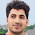# date input field on a visualforce

So as to show a date field(with a calender pop up similar to standard date input on standard record edit page) as input on a visualforce we need to make sure that the visualforce inputfield component is binded to a salesforce object field.
For this we can create a dumy object insatnce. We need to make sure the date field which we are going to find be defined in the variable insatnce.
If the visualforce page uses standard controller then we can directly bind using the object field as in the example below:

Visuaklforce page

If it is not a standard controller then we will need to create adumy instance of a object and define a date field as shown in the example below:
Visualforce Page
Controller

1.good example dear

2.This will work if you have a predefined date field in controller. What if you want to provide two date fields and return the records created between those two date values

3.Nice Example

4.Hi, Where is code for that, i see that in many examples, code is not there